TL;DR: This is an informal summary of our recent paper Walking in the Shadow: A New Perspective on Descent Directions for Constrained Minimization by Hassan Mortagy, Swati Gupta, and Sebastian Pokutta, where we present a novel theoretical framework for unifying and analyzing various descent directions considered in first-order optimization methods. Some of these descent directions include Frank-Wolfe, away steps and pairwise directions, which have been an important design consideration in conditional gradient descent (CGD) variants. We use our theoretical framework to develop algorithms with faster and more robust convergence rates than those existing in the literature.

Written by Hassan Mortagy.

## What is the paper about and why you might care?

We consider the problem

$\tag{Pr} \min_{ x \in P} f( x ),$

where $f: P \to \mathbb{R}^n$ is an $L$-smooth and $\mu$-strongly convex function, and $P\subseteq \mathbb{R}^n$ is a polytope. Smooth convex optimization problems over polytopes are an important class of problems that appear in many settings, such as low-rank matrix completion, structured supervised learning, video co-localization in computer vision and submodular function. First-order methods in convex optimization rely on movement in the best local direction for descent (e.g., negative gradient), and this is enough to obtain linear convergence for unconstrained optimization. In constrained settings however, the gradient may no longer be a feasible direction of descent, and there are two broad classes of methods traditionally: projection-based methods (i.e., move in direction of negative gradient, but project to ensure feasibility), and conditional gradient methods (i.e., move in feasible directions that approximate the gradient). Projection-based methods such as projected gradient descent or mirror descent enjoy dimension independent linear rates of convergence (assuming no acceleration), i.e., $(1-\frac{\mu}{L})$ contraction in the objective per iteration (so that the number of iterations to get an $\epsilon$-accurate solution is $O(\frac{L}{\mu} \log \frac{1}{\epsilon})$), but need to compute an expensive projection step (another constrained convex optimization) in (almost) every iteration.

On the other hand, conditional gradient methods (such as the Frank-Wolfe algorithm [FW] need to solve linear optimization (LO) problems in every iteration and the rates of convergence become dimension-dependent, for e.g., the away-step Frank-Wolfe algorithm has a linear rate of $(1-\frac{\mu \delta^2}{LD^2})$, where $\delta$ is a geometric constant (polytope dependent) and $D$ is the diameter of the polytope [LJ]. Moreover, descent directions such as movement towards Frank-Wolfe vertices, away steps, in-face away steps and pairwise directions have been an important design consideration in conditional gradient descent (CGD) variants. A natural question at this point is why are these different descent directions useful and which of these are necessary for linear convergence. In this paper, we attempt to demystify the impact of movement in these directions towards attaining constrained minimizers. If one had oracle access to the “best” local direction of descent for constrained minimization, what would it be and is it enough to get linear convergence (as in unconstrained optimization)? Moreover, can we avoid rates of convergence that are dependent on the geometry of the polytope? We partially answer these questions through our parametric projections framework, where our analysis develops properties of directional derivatives of projections (which may be of independent interest), while providing a unifying view of various descent directions in the CGD literature.

We let $\Pi_P(y) := \text{arg min}_{x \in P} \frac{1}{2} \|x - y\|_2^2$ to be the Euclidean projection operator over $P$. For any point $x \in P$ and direction $\mathbf{w} \in \mathbb{R}^n$ we define the shadow of the gradient as

$\mathbf{d}_x^\Pi (\mathbf{w}) \doteq \lim_{\epsilon \downarrow 0} \frac{\Pi_P(x - \epsilon \mathbf{w}) - x}{\epsilon}, \tag{Shadow}$

which is the directional derivative of the projection operator. When $\mathbf{w} = \nabla f(x)$, then we call $\mathbf{d}_{ x }^\Pi(\nabla f(x))$ the shadow of the gradient at $x$, and use notation $\mathbf{d}_{ x }^\Pi$ for brevity. This limit quantity has been looked at before in the literature. In particular, [MT] show that $\mathbf{d}_{ x }^\Pi$ is the projection of $-\nabla f(\mathbf x )$ onto the set of feasible directions at $x$, that is $\mathbf{d}_{ x }^\Pi = \text{arg min}_{\mathbf{d} \in \text{Cone}(P-x)} \|-\nabla f( x ) - \mathbf{d} \|^2$, where the uniqueness of the solution follows from strong convexity of the objective. We give it the name shadow because it is literally the shadow of the gradient onto the polytope.

Why is the shadow of the gradient important? We show that the shadow is the locally the optimal direction for descent. That is, for all $x \in P$ and feasible directions $\mathbf{y} \in \text{Cone}(P-x)$ we have

$\begin{equation} \label{best local direction equation} \left \langle -\nabla f( x ),\frac{\mathbf{d}_{ x }^\Pi}{\|\mathbf{d}_{ x }^\Pi\|} \right \rangle = \|\mathbf{d}_{\mathbf x }^\Pi \| \geq \left \langle-\nabla f( x ),\frac{\mathbf{y}}{ \|\mathbf{y}\|} \right \rangle. \tag{OptShadow} \end{equation}$

In unconstrained optimization we know that the negative gradient is locally the direction of steepest descent. However, in con stained settings, that gradient may no longer be feasible. Projection-based methods use projections to ensure feasibility, while conditional gradient methods use linear-optimization to use feasible directions that are reasonably aligned with the gradient. However, the shadow is the analog of that negative gradient in constrained optimization and its the direction that one really wants to move along.

## The Shadow and Parametric Projections Framework

We now present our Shadow and Parametric Projections Framework, which is central to the analysis of our algorithms and unification of first-order methods. For any point $x_0 \in P$ and direction $w \in \mathbb{R}^n$ we define $g(\lambda) = \Pi_P(x_0 - \lambda \mathbf{w})$ to be the parametric projections curve that is parameterized by the step-size $\lambda \in \mathbb{R}$. In the paper, we show that $g(\lambda)$ is a piecewise linear curve, where the breakpoints of the curve occur at points where there is a change in the normal cone. Let us now explain this with an example. First, we use $N_P(x)$ to denote the normal cone at $x\in P$. Consider the following example: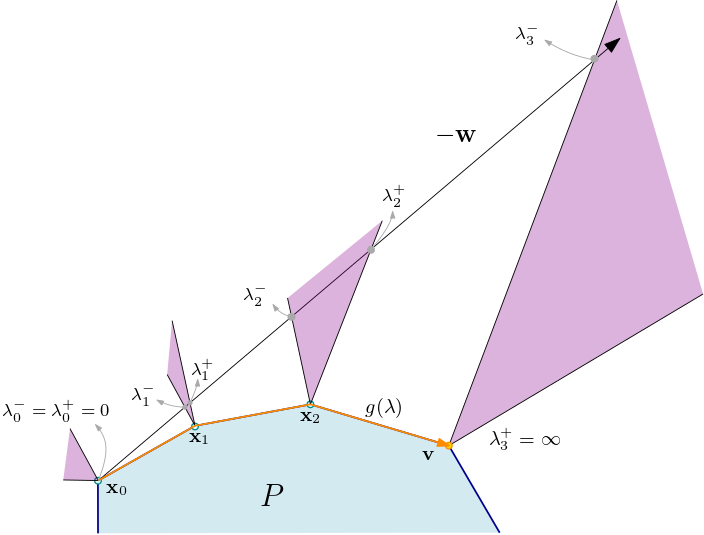Figure 1. Normal cones occurring along the projection curve.

In the above figure, the curve $g(\lambda)$ is depicted by the orange line and is piecewise linear with breakpoints $x_i$. First, $g(\lambda) = x_0 + \lambda {\mathbf{d}_x^\Pi(\mathbf{w})}$ for $\lambda \in [0,\lambda_1^{-}]$, where $\mathbf{d}_x^\Pi(\mathbf{w}) = \frac{x_1 - x_0}{\lambda_1^{-}}$ is the shadow with respect to $-\mathbf{w}$. At that point, we see that $x_0 - \lambda \mathbf{w} - x_1 \in N_P(x_1)$ for all $\lambda \in (\lambda_1^{-}, \lambda_1^{+}]$. Hence, $g(\lambda) = x_1$ for all $\lambda \in (\lambda_1^{-}, \lambda_1^{+}]$, i.e. we will keep projecting back to the same point $x_1$ in that interval. Thus, $g(\lambda)$ does not change at the same speed with respect to $\lambda$. Moreover, we have $N_P(g(\lambda)) = N_P(g(\lambda^\prime)) \subset N_P(x_0)$ for all $\lambda, \lambda^\prime \in (0,\lambda_1^{-})$. Then, another constraint becomes tight at the end point of the first segment $x_1$, and thus we have $N_P(g(\lambda)) = N_P(g(\lambda^\prime)) {\subset N_P(x_1)}$ for all $\lambda, \lambda^\prime \in (0,\lambda_1^{-})$. This process of adding and dropping constraints continues until we reach $\lambda_3^{-}$. We show that once the parametric projections curve (given by the orange line in the figure) leaves a face, it never returns to it again. At this point, $x_0 - \lambda \mathbf{w} - \mathbf{v} \in N_P(\mathbf{v})$ for $\lambda \geq \lambda_3^{-}$, i.e $g(\lambda) = \mathbf{v}$ and we will keep projecting back to $\mathbf{v}$. However, this implies that $\mathbf{v}$ if the FW vertex since $-\mathbf{w} \in N_P(\mathbf{v})$ if and only if $\mathbf{v} = \text{arg min}_{x \in P} \langle{\mathbf{w}}, x \rangle$. We will discuss this in more details later on.

Even though our structural results of $g(\lambda)$ hold for any direction $\mathbf{w}\in \mathbb{R}^n$, we will focus on the case when for $\mathbf{w} = \nabla f(x_0)$ for readability in the context of the paper. Using the previous discussion, we know that the curve $g(\lambda) = \Pi_P(x_0 - \lambda \nabla f(x_0))$ is piecewise linear with the first segment being the shadow of the gradient. Moreover, the number of breakpoints of that curve are at most the number of the faces of $P$. Another nice we show is that we constructively trace the entire projections curve using iterative line-search and shadow computations as follows: Given a breakpoint $x_{i-1}$ of $g(\lambda)$, to compute the subsequent breakpoint $x_i$ we just need to consider the gradient at $x_0$ and compute the shadow at $x_{i-1}$ with respect to that the original gradient (note that we are not changing the gradient) and then do a line-search for feasibility in that direction. More formally,

$\begin{equation} \label{trace} x_{i} = x_{i-1} + \delta^{\max} \mathbf{d}_{x_{i-1}}^\Pi (\nabla f(x_0)) \quad \text{for } \delta^{\max} = \max\{\delta \mid x_{i-1} + \delta^{\max} \mathbf{d}_{x_{i-1}}^{\Pi} (\nabla f(x_0)) \in P\}. \tag{Trace} \end{equation}$

Observe that to trace that entire projections curve, we just need to make one gradient oracle call.

## Descent Directions

We will now use our Shadow and Parametric Projections Framework to unify various results in first-order methods:

1. (PGD) It is easy to see that multiple line searches in the directional derivatives with respect to $x_0$ are equivalent to computing a single projected gradient descent (PGD) step from $x_0$, since by construction the PGD step lies on the projections curve which we know how to trace.

2. (FW Steps) We also show that the same holds true for FW vertices. In particular, when the FW vertices are unique they form the endpoint of the projections curve given by $\lim_{\lambda \to \infty} g(\lambda)$. Projected gradient (steps) provide a contraction in the objective independent of the geometric constants or facets of the polytope; they are also able to “wrap” around the polytope by taking unconstrained gradient steps and then projecting. Thus, the Frank-Wolfe vertices are in fact the projection of an infinite descent in the negative gradient direction. This allows the CG methods to wrap around the polytope maximally, compared to PGD methods, thereby giving FW steps a new perspective.

3. (Shadow steps). As previously mentioned, the shadow of the gradient is locally the optimal direction of descent $\eqref{best local direction equation}$. Shadow steps also give a true estimate of convergence to optimal, in the sense that $\|\mathbf{d}_{\mathbf x } ^\Pi\| = 0$ if and only if $x = \arg\min_{x\in P} f(x)$. On the other hand, note that $\|\nabla f(x)\|$ does not satisfy this property and can be strictly positive at the constrained optimal solution. Furthermore, the shadow can be used as a tight proxy for the primal gap for strongly-convex functions without any dependence on geometric constants: $\|\mathbf{d}_ x ^\Pi \|^2 \geq 2 \mu (f(x) - f(x^*))$. Note that CG variants do not enjoy this property.

4. (Away steps): Shadow steps are the best normalized away-direction in the following sense: let $F$ be the minimal face containing the current iterate $x_t$ (similar to [GM] and [BZ]); then, $x _t - \gamma \mathbf{d}_{x_t}^\Pi \in \text{conv}(F)$ (i.e., the backward extension from $x_t$ in the shadow direction), and the resultant direction ( $\mathbf{d}_{x_t}^\Pi$) is indeed the most aligned with $-\nabla f(x_t)$ (using 1. above). Shadow-steps are, however, in general convex combinations of potential active vertices minus the current iterate and therefore loose combinatorial properties such as dimension drop in active sets.

5. (Pairwise steps): The progress in CG variants is bounded crucially using the inner product of the descent direction with the negative gradient. In this sense, pairwise steps are simply the sum of the FW step and away directions, and a simple algorithm that uses these steps only does converge linearly (with geometric constants) [LJ],[BZ]. Thus, we can also use our framework to obtain optimal pairwise steps.

Using our insights on descent directions, we propose the $\textsf{Shadow-CG}$ algorithm, which combines FW steps and shadow steps. We give an example below: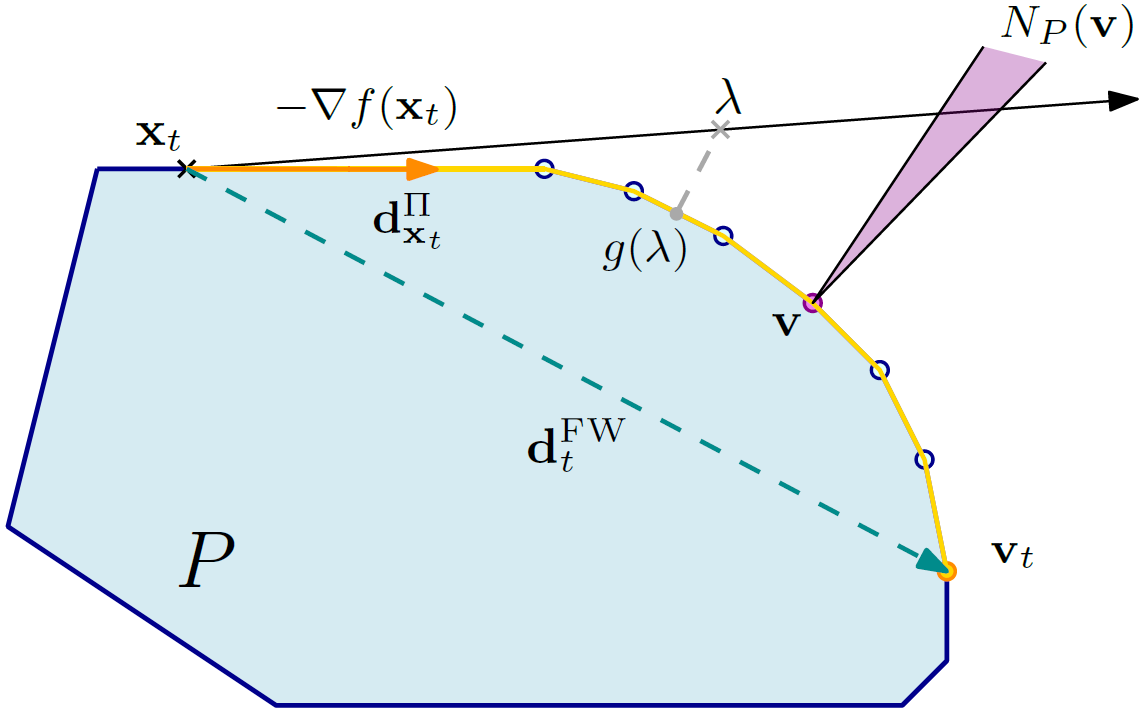Figure 2. Projection curve and shadows.

In the figure above the projections curve $g(\lambda)$ is the yellow curve whose first segment is the shadow at $x_t$. In each iteration we either take the FW step (which is the green direction in the above figure), or we trace the projections curve using our constructive procedure of iterative shadow and line-search computations $\eqref{trace}$ until we have sufficient progress. The idea is that Frank-Wolfe steps allow us to greedily skip a lot of facets by wrapping maximally over the polytope. This prevents us from tracing the projections curve every iteration, which can be expensive. Moreover, shadow steps operate as “optimal” away-steps (4. above) thus reducing zig-zagging phenomenon close to the optimal solution. As the algorithm progresses, one can expect Frank-Wolfe directions to become close to orthogonal to negative gradient. However, in this case the norm of the shadow also starts diminishing. Therefore, we choose FW direction whenever $\langle {-\nabla f(x_{t})},{ \mathbf{d}_t^{\text{FW}}} \rangle \geq \langle{-\nabla f(x_t)},{ { \mathbf{d}_{x_t}^\Pi}/{\|\mathbf{d}_{x_t}^\Pi\|}} \rangle = \|\mathbf{d}_{x_t}^\Pi\|$, and the trace procedure otherwise. This is sufficient to give linear convergence without any dependence on geometric constants:

Theorem (informal). The primal gap $h( x _t): = f( x _t) - f( x ^*)$ of $\textsf{Shadow-CG}$ decreases geometrically: $h( x _{t+1}) \leq \left(1- \frac{\mu}{ LD^2}\right)h( x _t),$ with each iteration of the $\textsf{Shadow-CG}$ algorithm (assuming the trace procedure is a single step). Moreover, the number of shadow and line oracle calls for an $\epsilon$-accurate solution is $O\left((D^2+ \beta) \frac{L}{\mu} \log (\frac{1}{\epsilon}) \right)$, where $\beta$ is the number of breakpoints of the parametric projections curve that the trace procedure visits.

Although the constant $\beta$ depends on the number of faces in the worst-case, and in fact the combinatorial structure of the face-lattice of the polytope, it is invariant under any deformations of the actual geometry of the polytope preserving the face-lattice (in contrast to vertex-facet distance [GH] and pyramidal width [LJ]). Therefore, we smoothly interpolate between the $\left(1 - \frac{\mu}{L} \right)$ rate for PGD and the rates for CG variants.

Garber and Meshi [GM] and Bashiri and Zhang [BZ] both compute the best away vertex in the minimal face containing the current iterate, whereas the shadow step recovers the best convex combination of such vertices aligned with the negative gradient. Therefore, these previously mentioned CG methods can both be viewed as approximations of $\textsf{Shadow-CG}$.

## Computations

We consider the video co-localization problem from computer vision, where the goal is to track an object across different video frames. We used the YouTube-Objects dataset [LJ] and the problem formulation of Joulin et. al [JTF]. This consists of minimizing a quadratic function $f(x) = \frac{1}{2}x^TAx + \mathbf{b}^T x$, where $x \in \mathbb{R}^{660}$, $A \in \mathbb{R}^{660 \times 660}$ and $\mathbf{b}\in \mathbb{R}^{660}$, over a flow polytope, the convex hull of paths in a network.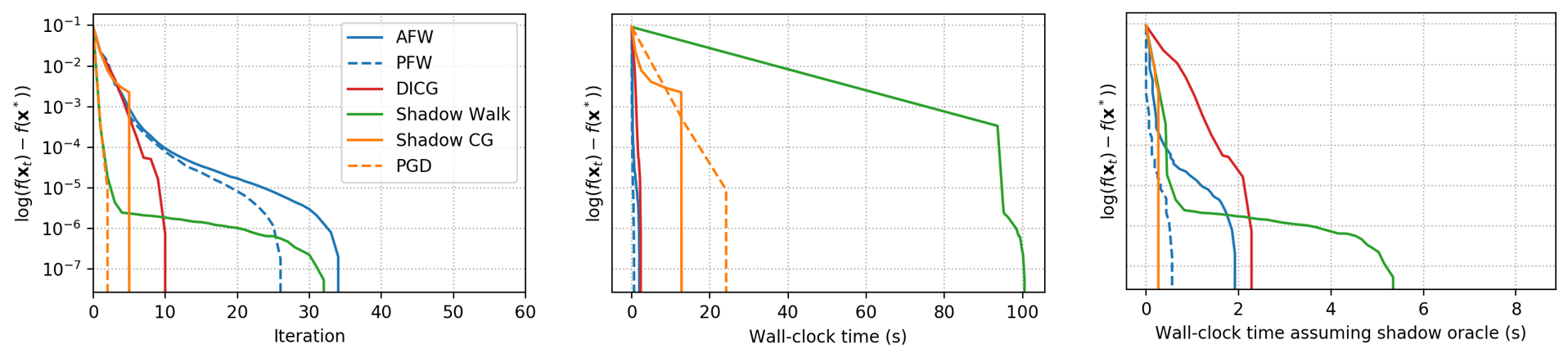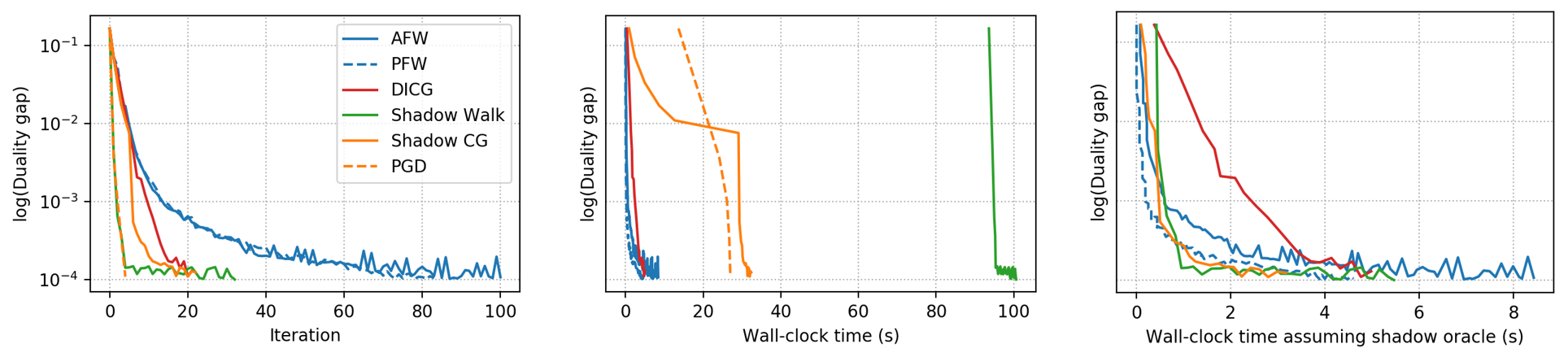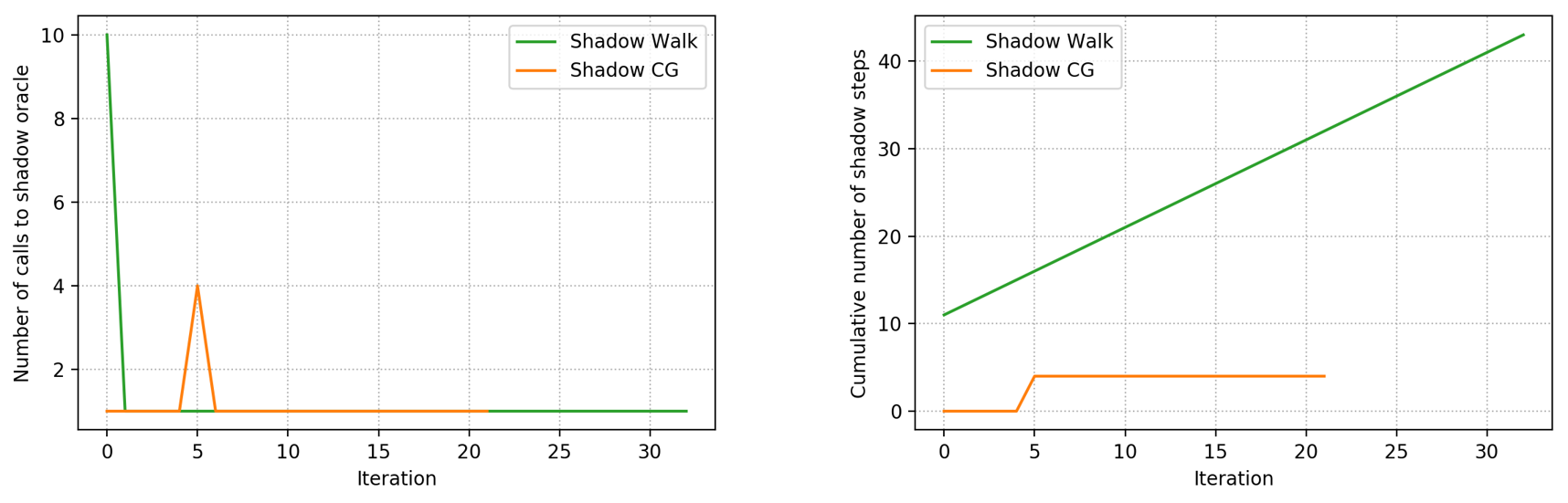Figure 3. Computational results on video co-localization. Top: log primal gap. Middle: log dual gap. Bottom: number of calls to shadow oracle

We observe above that $\textsf{Shadow-CG}$ has lower iteration count than CG variants (slightly higher than PGD), while also improving on wall-clock time compared to PGD (i.e., close to CG) without assuming any oracle access, thus our algorithms interpolate between CG variants and PGD. Moreover, when assuming access to shadow oracle, $\textsf{Shadow-CG}$ outperforms the CG variants both in iteration count and wall-clock time. Finally, we observe that the number of iterations spent in $\textsf{Trace}$ is much smaller (bounded by 10 for $\textsf{Shadow-Walk}$ and by 4 for $\textsf{Shadow-CG}$) than the number of faces of the polytope. $\textsf{Shadow-CG}$ spends much fewer iterations in $\textsf{Trace}$ than $\textsf{Shadow-Walk}$ (which is an algorithm that just traces the projections curve every iteration and does not have FW steps) due to the addition of FW steps. We refer the reader to paper for additional computational results, with qualitatively similar findings.

[BZ] M. A. Bashiri and X. Zhang (2017). Decomposition-invariant conditional gradient for general polytopes with line search. In Proceedings of the 31st International Conference on Neural Information Processing Systems (pp. 2687–2697). pdf

[JTF] A. Joulin, K. D. Tang, and F. Li (2014). Efficient image and video co-localization with frank-wolfe algorithm. in Computer Vision - ECCV 2014 - 13th European Conference (pp. 253–258). pdf

[FW] M. Frank and P. Wolfe (1956). An algorithm for quadratic programming. Naval Research Logistics Quarterly, vol. 3, no. 1-2, (pp. 95–110) link

[LJ] Lacoste-Julien, S. & Jaggi, M. (2015). On the global linear convergence of Frank-Wolfe optimization variants. In Advances in Neural Information Processing Systems 2015 (pp. 496-504). pdf

[GH] D. Garber and E. Hazan, O.(2016). A linearly convergent variant of the conditional gradient algorithm under strong convexity, with applications to online and stochastic optimization. SIAM Journal on Optimization, vol. 26, no. 3 (pp. 1493–1528). pdf

[GM] Garber, D. & Meshi, O.(2016). Linear-memory and decomposition-invariant linearly convergent conditional gradient algorithm for structured polytopes. In Advances in Neural Information Processing Systems 2016 (pp. 1001-1009). pdf

[MT] G. P. McCormick and R. A. Tapia (1972). The gradient projection method under mild differentiability conditions. SIAM Journal on Control, vol. 10, no. 1. (pp. 93–98). link